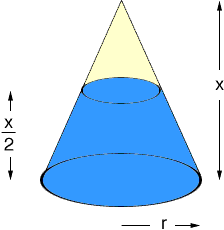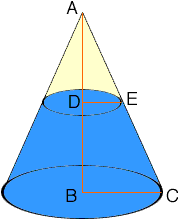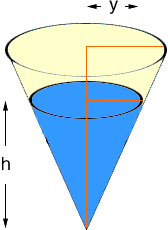Quandaries and Queries Hi I'm a grade 8 math teacher. There are 2 right cones, height is X. one of them is filled from the bottom up (round side) = x/2, when you invert the cone and add the same amount of liquid inside the second cone, what is the HEIGHT of the liquid? (thin side) you need 2 diagrams. Hi Vasuki, I drew a diagram of the cone filled to half the height and I labeled the radius of the base circle r.The volume of a cone is  1/3(radius of the base)2height so the volume of this cone is  1/3r 2x I am going to find the volume of liquid in the cone by subtracting the volume of the unfilled part of the cone. To do this I need to find the radius of the base of the unfilled part of the cone. This involves a use of similar triangles. In the diagram below triangles ABC and ADE are similar and hence  |AB|/|BC| = |AD|/|DE| or  x/r = (x/2)/|DE|Thus |DE| = r/2 Thus the volume of liquid in the cone is  1/3r 2x -  1/3(r/2)2x =  1/4r 2x Now turn the cone over.Now I have labeled the height of the liquid h and the radius of the circle at the top of the liquid y. Thus the volume of the liquid is  1/3y 2h But the volume of the liquid hasn't changed so  1/3y 2h =  1/4r 2x Again using similar triangles, this time from the diagram above  y/h = r/x so y = (rh)/x Substitute this value of y into  1/3y 2h =  1/4r 2x and solve for h. I got the cube root of 3/4, times x. Penny Go to Math Central